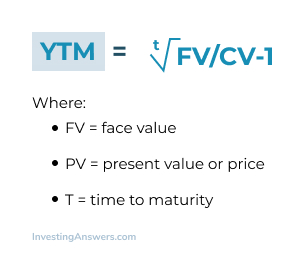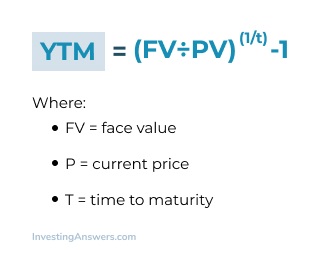# Yield to Maturity (YTM)

Updated July 30, 2021

## What Is Yield to Maturity (YTM)?

Also referred to as book yield and redemption yield, yield to maturity (YTM) is the total return that’s anticipated on a bond or other fixed-price security. The YTM is based on the assumption that the investor will purchase a bond and hold it until maturity. It also assumes that  all coupon payments are reinvested during the same period at the same rate.

### Why Yield to Maturity Is Useful

Yield to maturity helps investors make comparisons between the types of returns they can expect from different securities. Doing so helps them determine which types of securities to include in their investment portfolio.

YTM also lets investors understand how market changes may affect their investments when yields rise or drop in price.

## Is Yield to Maturity the Same as Coupon Rate?

The yield to maturity is the percentage of the rate of return for a fixed-rate security should an investor hold onto the asset until maturity. The coupon rate is simply the amount of interest an investor will receive. Also known as nominal yield or the yield from the bond, the coupon rate doesn’t change.

Simply put, it is the total value of all coupon payments (or the annual interest rate of the security) plus any gain (loss) in value as a percentage of the initial investment. The YTM can rise or fall, depending on factors such as the number payments until maturity and the bond’s market value.

## The Difference Between Yield Maturity Rate and Interest Rate?

Yield to maturity refers to the return (or yield) that an investor will earn from their investment, which is typically reported as an annual rate. The return is comprised of interest payments (referred to as coupons) and any gain in the bond’s market value. The yield is based on the coupon rate the bondissuer agrees to pay.

The interest rate is the same as the coupon rate. It represents the regular payments based on the principal amount that an investor used to purchase the bond.

## Types of Yield to Maturity

There are several types of YTM calculations that are more appropriate, depending on the fixed-price security an investor is analyzing.

### Yield to Call vs. Yield to Maturity

Calculating yield to maturity requires an underlying assumption that all interest payments are paid and reinvested at the same rate until the bond reaches maturity. It’s based on the coupon rate, purchase price, years until maturity, and the bond’s face value.

A yield to call (YTC) is the total return that an investor will receive if the bond is held until the call date (or whenever the bond issuer has the right to redeem it). This date is usually before the full maturity date. The YTC is calculated based on the time until the call date, the market price, and the bond’s coupon rate.

### Yield to Call vs. Yield to Worst

The yield to worst is sometimes referred to as the yield to call. Yield to worst (YTW) refers to the worst possible yield for a bond without the bond issuer going into default. Think of it as the “worst-case scenario” for your investment.

Some of these scenarios include anything that would negatively impact the yield, like provisions that lower the coupon rate based on market conditions. YTW also assumes that there won’t be any provisions or recalculations in favor of investors.

### Yield to Call vs. Yield to Put

Yield to call is the yield that an investor can receive by holding the bond until the call date. Investors tend to receive a higher coupon rate since the bond issuer has the option of calling it back above the predetermined price.

On the other hand, yield to put is what the investor can yield when holding the bond until they sell it back to the issuer. Investors tend to receive a lower coupon rate since they’re able to sell bonds back to the bond issuer and reinvest their funds elsewhere (at potentially higher rates).

## The formula to calculate YTM is as follows:Yield to Maturity Example

Let’s say you’re thinking about purchasing a bond that’s priced at \$1,000 and has a face value of \$1,500. The bond will mature in 6 years and the coupon rate is 5%.

To determine the YTM, we’ll use the formula mentioned above:

YTM =  t√\$1,500/\$1,000 - 1

The estimated YTM for this bond is 13.220%.

## How Yield to Maturity Is Calculated (for Zero Coupon Bonds)

Since zero coupon bonds don’t have recurring interest payments, they don’t have a coupon rate.

The zero coupon bond formula is as follows:For instance, you want to invest in a \$1,000 zero coupon bond that has three years until it matures. It’s currently valued at \$1,825. Plugging in these numbers using the formula above, it’ll produce a value of 0.0954004597 (or a yield of 9.54%).

## How to Calculate Yield to Maturity with a Financial Calculator

To use a financial calculator to calculate YTM, you’ll need information about:

• The bond’s current price

• Years to maturity

• Face value

• Coupon rate

These details will then be entered in the financial calculator’s time value of money functions. You’ll then solve for interest (I), which will provide you the yield to maturity.

## How to Calculate Yield to Maturity in Excel

Using the YTM formula will only give you an approximation. While that’s useful, to determine a true YTM, investors need to use trial and error. This process works by using different coupon rates to calculate possible yields. You’ll be able to find the true YTM when the price you calculated matches the bond’s actual current market price.

To speed this process up, you can use the SOLVER function in Excel to determine a value based on a set of conditions. This means that you can set the price (present value) for the bond and solve for the yield to maturity (the interest rate for the present value calculation).

## Can Yield to Maturity Be Negative?

Yes, but for this to happen, the bond must have a negative total return. That’s because the calculation for YTM includes the payout when the bond matures. For a negative YTM to occur, the bond would need to sell at a price so high that any future coupon/interest payments couldn’t make up for the initial investment.

## Advantages of Yield to Maturity

A major advantage of yield of maturity is that it factors in  cash flow expected to be generated in the future. Other additional advantages include YTM:

• Considering both revenue and capital gains, thus showing a more complete earnings picture

• Providing more accurate information since YTM does not include any forecasts in the calculation

• Calculating the total return an investor can earn during bond’s lifetime

## Disadvantages of Yield to Maturity

Although YTM is useful, there are a few downsides such as:
Assuming the bond will be held until the maturity date
Unrealistically assuming that all earnings are reinvested at the same rate.

All of our content is verified for accuracy by Rachel Siegel, CFA and our team of certified financial experts. We pride ourselves on quality, research, and transparency, and we value your feedback. Below you'll find answers to some of the most common reader questions about Yield to Maturity (YTM).

## What Happens When YTC Increases?

The YTC typically moves in the same direction as the YTM with some differences. If a bond trades at a lower or discount price than the future value, the YTC will be higher than the nominal yield and YTM. If it trades for a premium price, the YTC will be lower than the YTM and nominal yield. If this happens, the bond will face a loss when it matures.

## Why Are Yield to Maturity and Price Inversely Related?

When interest or coupon rates on new bond issues go up, existing bonds become less attractive and the prices of existing bonds will fall, which increases their YTMs

When rates fall, the opposite happens: Prices go up until the yield of older bonds is low enough to match rates on newer bond issues. Think of it as a way to ensure there is still demand to purchase bonds by altering its price in response to yield changes.

## Is Yield to Maturity the Same as Discount Rate?

Yes, the YTM can be viewed as a discount rate, which, when applied, makes the value of the future cash flows equal to the bond’s current market price.

## What Happens When YTC Increases?

The YTC typically moves in the same direction as the YTM with some differences. If a bond trades at a lower or discount price than the future value, the YTC will be higher than the nominal yield and YTM. If it trades for a premium price, the YTC will be lower than the YTM and nominal yield. If this happens, the bond will face a loss when it matures.

## Why Are Yield to Maturity and Price Inversely Related?

When interest or coupon rates on new bond issues go up, existing bonds become less attractive and the prices of existing bonds will fall, which increases their YTMs

When rates fall, the opposite happens: Prices go up until the yield of older bonds is low enough to match rates on newer bond issues. Think of it as a way to ensure there is still demand to purchase bonds by altering its price in response to yield changes.

## Is Yield to Maturity the Same as Discount Rate?

Yes, the YTM can be viewed as a discount rate, which, when applied, makes the value of the future cash flows equal to the bond’s current market price.

Rachel Siegel, CFA - 141Rachel Siegel, CFACFA Charterholder

Chartered Financial Analyst

Rachel Siegel, CFA is one of the nation's leading experts at ensuring the accuracy of financial and economic text.  Her prestigious background includes over 10 years creating professional financial certification exams and another 20 years of college-level teaching.